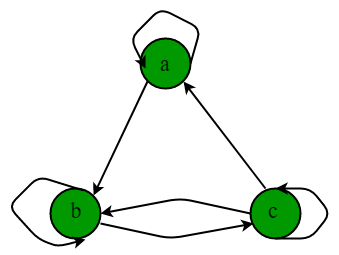# Mathematics | Representations of Matrices and Graphs in Relations

• Difficulty Level : Easy
• Last Updated : 28 Jun, 2021

Previously, we have already discussed Relations and their basic types.
Combining Relation:
Suppose R is a relation from set A to B and S is a relation from set B to C, the combination of both the relations is the relation which consists of ordered pairs (a,c) where a Є A and c Є C and there exist an element b Є B for which (a,b) Є R and (b,c) Є S. This is represented as RoS.

Inverse Relation:
A relation R is defined as (a,b) Є R from set A to set B, then the inverse relation is defined as (b,a) Є R from set B to set A. Inverse Relation is represented as R-1

R-1 = {(b,a) | (a,b) Є R}.

Complementary Relation:
Let R be a relation from set A to B, then the complementary Relation is defined as- {(a,b) } where (a,b) is not Є R.

Representation of Relations:
Relations can be represented as- Matrices and Directed graphs.

Relation as Matrices:
A relation R is defined as from set A to set B,then the matrix representation of relation is MR= [mij] where

mij = { 1, if (a,b) Є R

0, if (a,b) Є R }

Properties:

1. A relation R is reflexive if the matrix diagonal elements are 1.2. A relation R is irreflexive if the matrix diagonal elements are 0.
3. A relation R is symmetric if the transpose of relation matrix is equal to its original relation matrix. i.e. MR = (MR)T4. A relation R is antisymmetric if either mij = 0 or mji =0 when i≠j.
5. A relation follows join property i.e. the join of matrix M1 and M2 is M1 V M2 which is represented as R1 U R2 in terms of relation.
6. A relation follows meet property i.r. the meet of matrix M1 and M2 is M1 ^ M2 which is represented as R1 Λ R2 in terms of relation.

Relations as Directed graphs:

A directed graph consists of nodes or vertices connected by directed edges or arcs. Let R is relation from set A to set B defined as (a,b) Є R, then in directed graph-it is represented as edge(an arrow from a to b) between (a,b).

Properties:

1. A relation R is reflexive if there is loop at every node of directed graph.
2. A relation R is irreflexive if there is no loop at any node of directed graphs.
3. A relation R is symmetric if for every edge between distinct nodes, an edge is always present in opposite direction.
4. A relation R is asymmetric if there are never two edges in opposite direction between distinct nodes.
5. A relation R is transitive if there is an edge from a to b and b to c, then there is always an edge from a to c.

Example:

The directed graph of relation R = {(a,a),(a,b),(b,b),(b,c),(c,c),(c,b),(c,a)} is represented as :Since, there is loop at every node,it is reflexive but it is neither symmetric nor antisymmetric as there is an edge from a to b but no opposite edge from b to a and also directed edge from b to c in both directions. R is not transitive as there is an edge from a to b and b to c but no edge from a to c.